# Lesson 59 Linear Differential Equations IBHL Calculus Santowski

• Slides: 13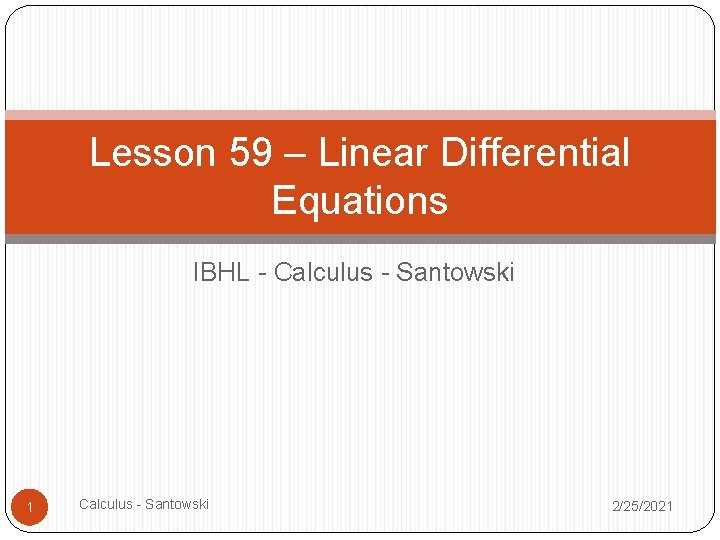Lesson 59 – Linear Differential Equations IBHL - Calculus - Santowski 1 Calculus - Santowski 2/25/2021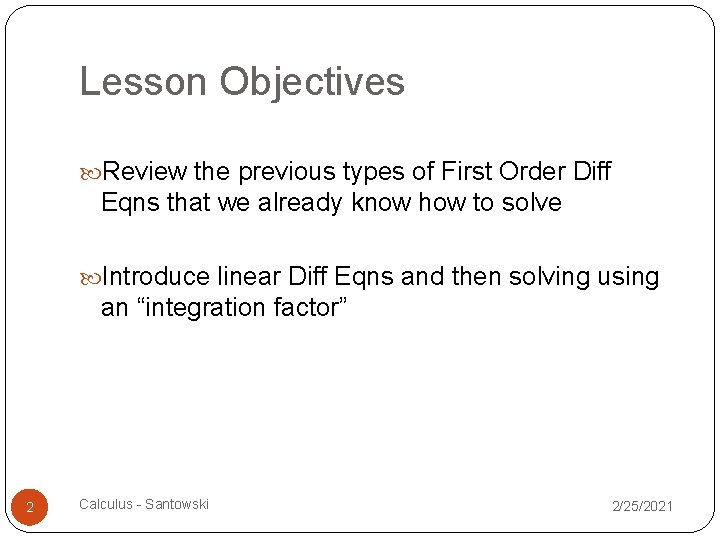Lesson Objectives Review the previous types of First Order Diff Eqns that we already know how to solve Introduce linear Diff Eqns and then solving using an “integration factor” 2 Calculus - Santowski 2/25/2021(A) Linear Diff Eqns Consider the following Diff Eqns. Two are linear Diff Eqns and two are not 3 Calculus - Santowski 2/25/2021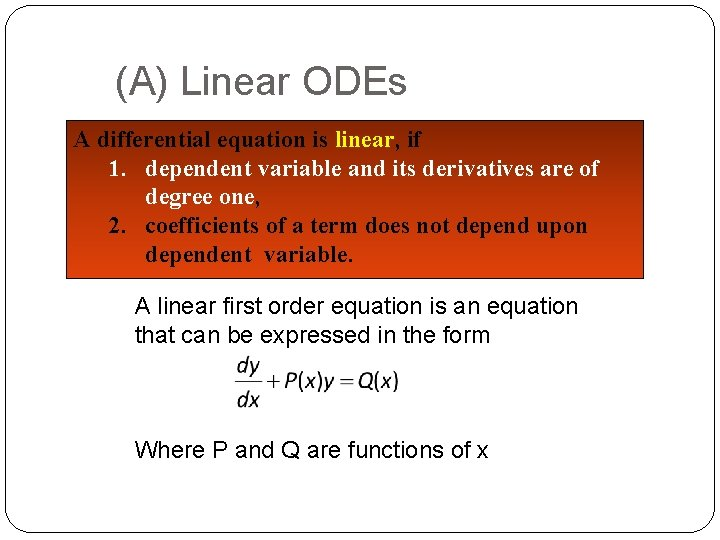(A) Linear ODEs A differential equation is linear, if 1. dependent variable and its derivatives are of degree one, 2. coefficients of a term does not depend upon dependent variable. A linear first order equation is an equation that can be expressed in the form Where P and Q are functions of x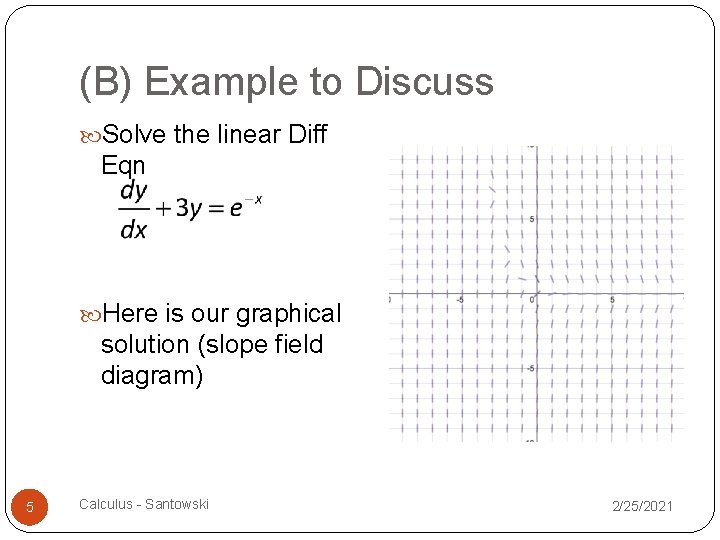(B) Example to Discuss Solve the linear Diff Eqn Here is our graphical solution (slope field diagram) 5 Calculus - Santowski 2/25/2021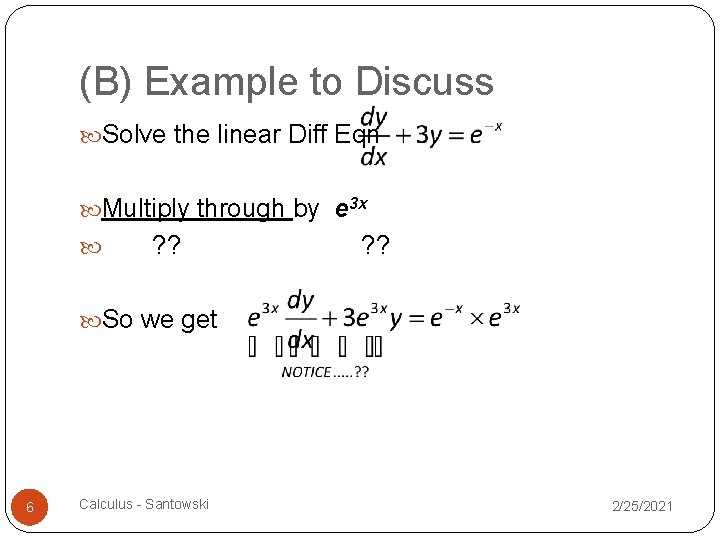(B) Example to Discuss Solve the linear Diff Eqn Multiply through by e 3 x ? ? So we get 6 Calculus - Santowski 2/25/2021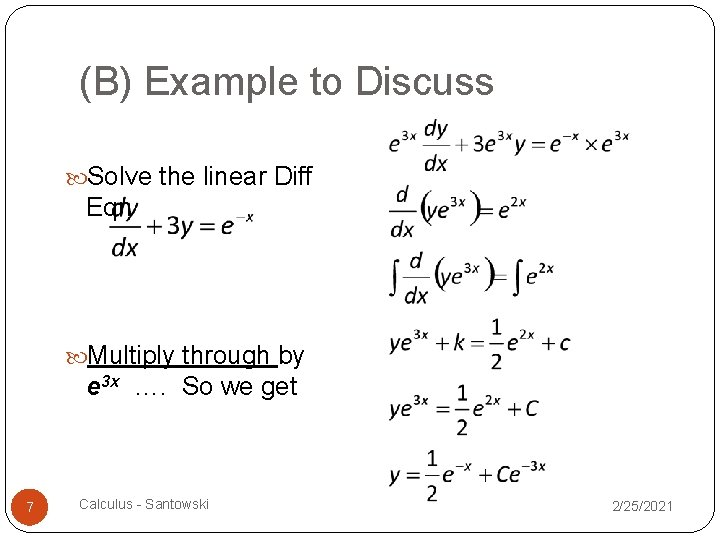(B) Example to Discuss Solve the linear Diff Eqn Multiply through by e 3 x …. So we get 7 Calculus - Santowski 2/25/2021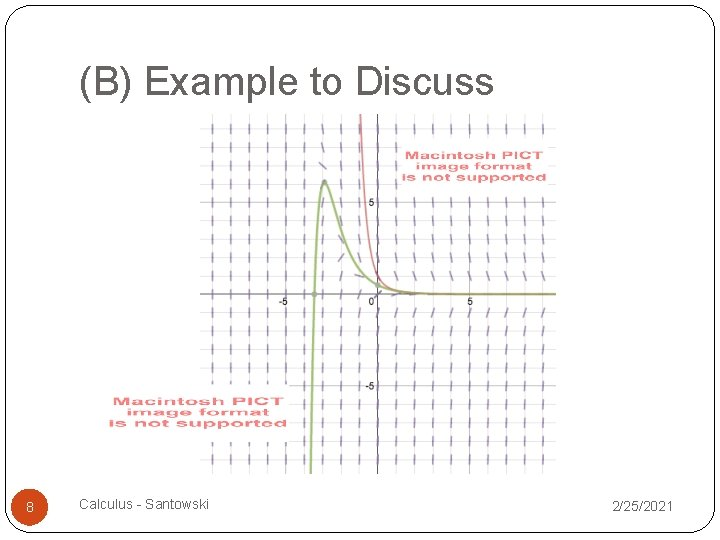(B) Example to Discuss 8 Calculus - Santowski 2/25/2021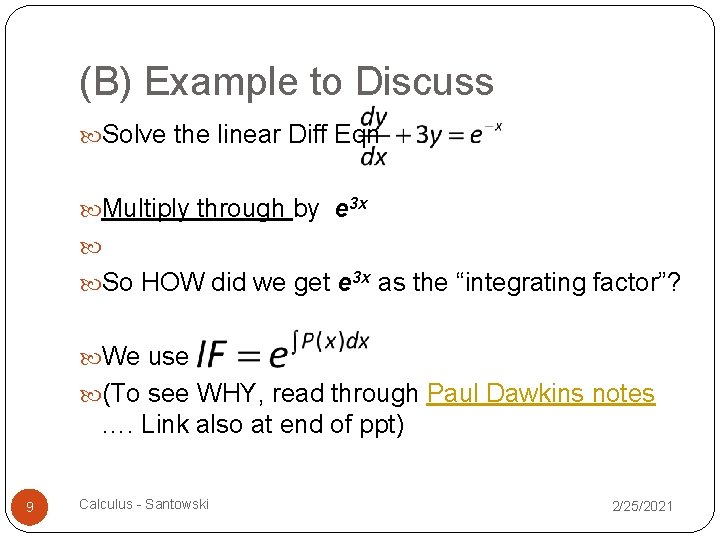(B) Example to Discuss Solve the linear Diff Eqn Multiply through by e 3 x So HOW did we get e 3 x as the “integrating factor”? We use (To see WHY, read through Paul Dawkins notes …. Link also at end of ppt) 9 Calculus - Santowski 2/25/2021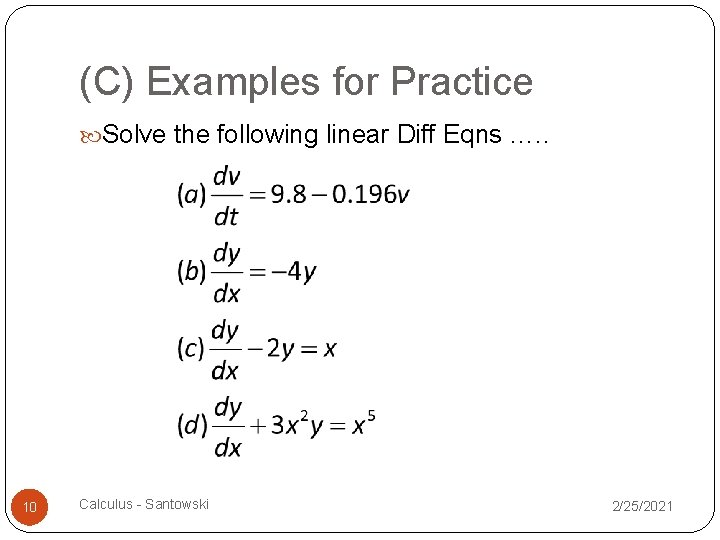(C) Examples for Practice Solve the following linear Diff Eqns …. . 10 Calculus - Santowski 2/25/2021(D) Further Examples for Practice Solve the following linear Diff Eqns …. . 11 Calculus - Santowski 2/25/2021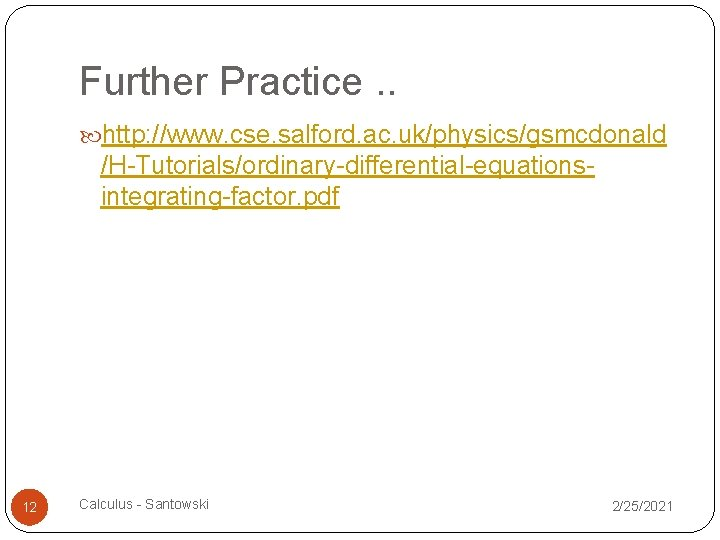Further Practice. . http: //www. cse. salford. ac. uk/physics/gsmcdonald /H-Tutorials/ordinary-differential-equationsintegrating-factor. pdf 12 Calculus - Santowski 2/25/2021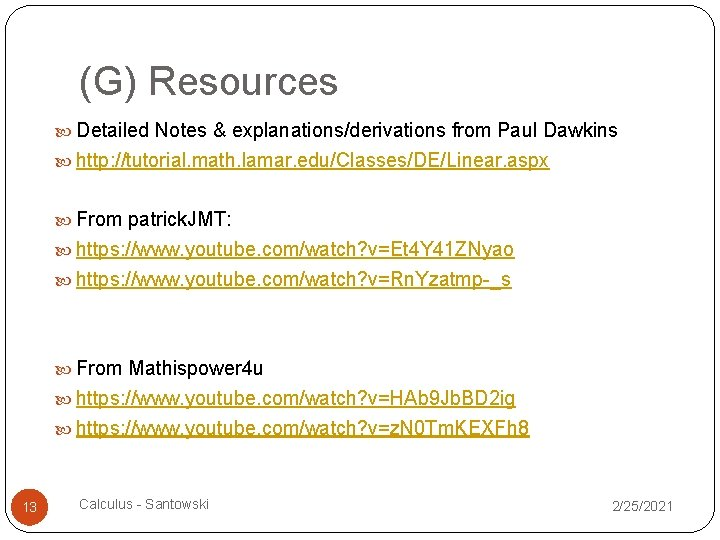(G) Resources Detailed Notes & explanations/derivations from Paul Dawkins http: //tutorial. math. lamar. edu/Classes/DE/Linear. aspx From patrick. JMT: https: //www. youtube. com/watch? v=Et 4 Y 41 ZNyao https: //www. youtube. com/watch? v=Rn. Yzatmp-_s From Mathispower 4 u https: //www. youtube. com/watch? v=HAb 9 Jb. BD 2 ig https: //www. youtube. com/watch? v=z. N 0 Tm. KEXFh 8 13 Calculus - Santowski 2/25/2021